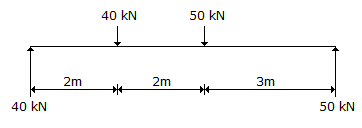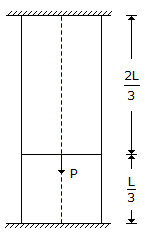# Civil Engineering - UPSC Civil Service Exam Questions

21.

What is the magnitude of the resultant of parallel force system as shown in the figure ?A. 70 kNm B. zero C. 70 KNm couple anti clock wise D. 90 KN veritical force and a moment of 70 kNm clock wise.

Explanation:

No answer description available for this question. Let us discuss.

22.

A rod shown in the given figure is such that the lower part is made of steel and the upper part is made of copper. Both the ends of the rod are rigidly clamped. If an axial force P is applied at the junction of the two metals, given that the ratio Esteel/ECopper is equal to 2, then the force in the copper rod would beA. P/5 tension B. P/3 tension C. P/3 compression D. P/5 compression

Explanation:

No answer description available for this question. Let us discuss.

23.

'Air binding' may occur in

 A. Sewers B. Artesian well C. Aerator D. Filter

Explanation:

No answer description available for this question. Let us discuss.

24.

For a vertical concentrated load acting on the surface of a semi-infinite elastic soil mass, the vertical normal stress at depth z is proportional to

 A. z B. z2 C. z3 D. 1/z2

Explanation:

No answer description available for this question. Let us discuss.

25.

Presence of nitrogen in waste water sample is due to the decomposition of

 A. Carbohydrates B. Proteins C. Fats D. Vitamins